• 多行公式
2022-06-08 20:07:16

在一行公式最右边按下 shift+ enter（软回车），即可转行。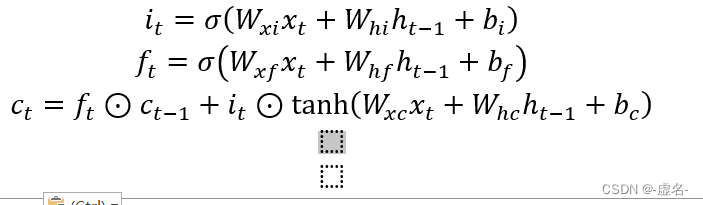windows
更多相关内容
• 提示： \begin{align} 与 \begin{equation}不能同时使用 \begin{aligned} 可以与 \begin{equation}同时使用 ...多行公式多编号 % 多行公式多编号 \begin{align} \hat{C}_t &= tanh(W_C\...

目录

多行公式多编号

多行公式单编号，编号居中

多行公式无编号

多行公式多编号，有的行没有编号

一行公式分多行写

情况（case）划分

大括号单编号

大括号多编号

提示：

• \begin{align} 与 \begin{equation}不能同时使用
• \begin{aligned} 只能与 \begin{equation}同时使用
• 要想使用\begin{align}，需要在 \begin{document} 之前插入\usepackage{amsmath}
• \begin{align}的用法应该与\begin{gather}用法相同，区别是：
• \begin{align}是默认右对齐，或者用 & 指定对齐位置
• \begin{gather} 默认是居中对齐，不能与 & 结合使用

概括：

• 导言区要加上，amsmath宏包
• 如果想有编号，可以用gather，align
• 如果想某一行没有编号，在用\\换行前使用\nonumber
• 如果不想有编号，可以gather*，align*，或者在后面加上命令\notag
• 如果想一行公式分开写，用split
• 如果想选择判断，用cases
• align，split，cases 的公式对齐，用&

这里主要介绍使用 \begin{align}的公式编号，以LSTM的公式为例子：

## 多行公式多编号

% 多行公式多编号
\begin{align}
\hat{C}_t &= tanh(W_C\odot[h_{t-1},x_t]+b_C) \\
i_t&=\sigma(W_i\odot[h_{t-1},x_t]+b_i) \\
f_t&=\sigma(W_f\odot[h_{t-1},x_t]+b_f) \\
C_t&=f_t * C_{t-1}+i_t * \hat{C}_t \\
o_t&=\sigma(W_o\odot[h_{t-1},x_t]+b_o) \\
h_t&=o_t * tanh(C_t)
\end{align}

实现效果：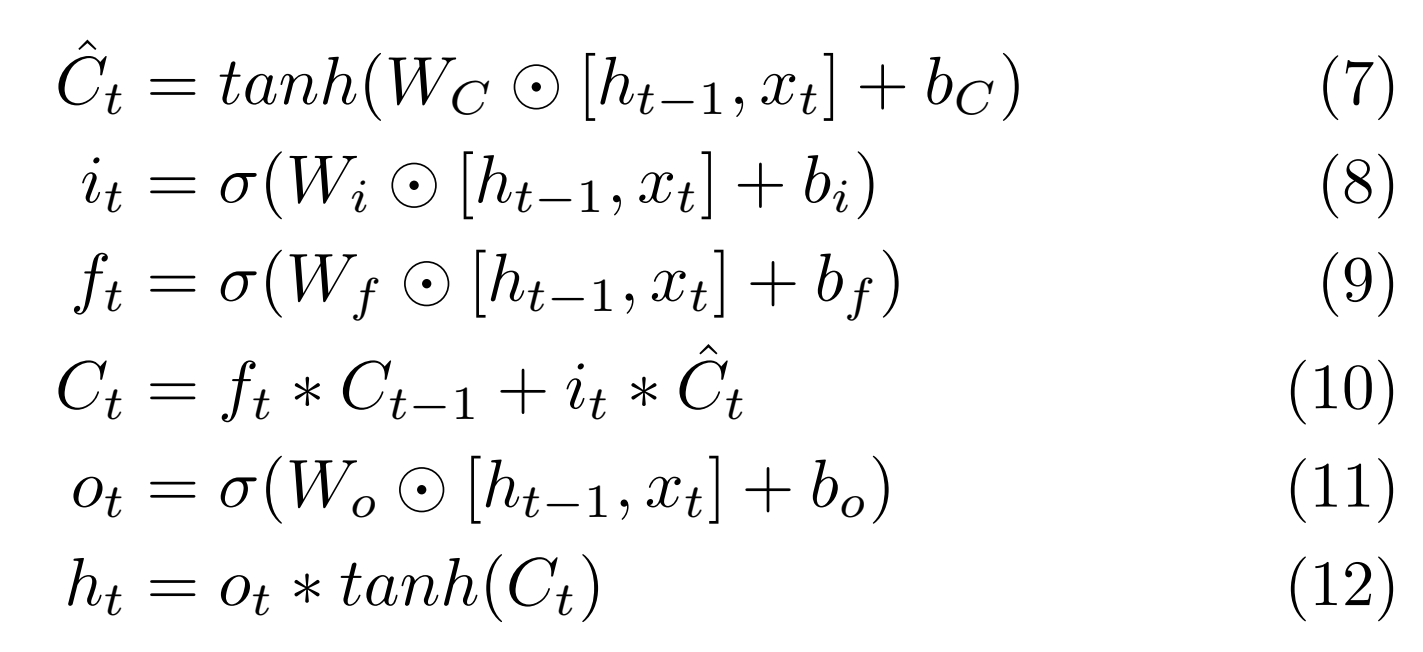## 多行公式单编号，编号居中

% 多行公式单编号
\begin{equation}
\begin{aligned}
&\hat{C}_t = tanh(W_C\odot[h_{t-1},x_t]+b_C) \\
&i_t=\sigma(W_i\odot[h_{t-1},x_t]+b_i) \\
&f_t=\sigma(W_f\odot[h_{t-1},x_t]+b_f) \\
&C_t=f_t * C_{t-1}+i_t * \hat{C}_t \\
&o_t=\sigma(W_o\odot[h_{t-1},x_t]+b_o) \\
&h_t=o_t * tanh(C_t)
\end{aligned}
\end{equation}

实现效果：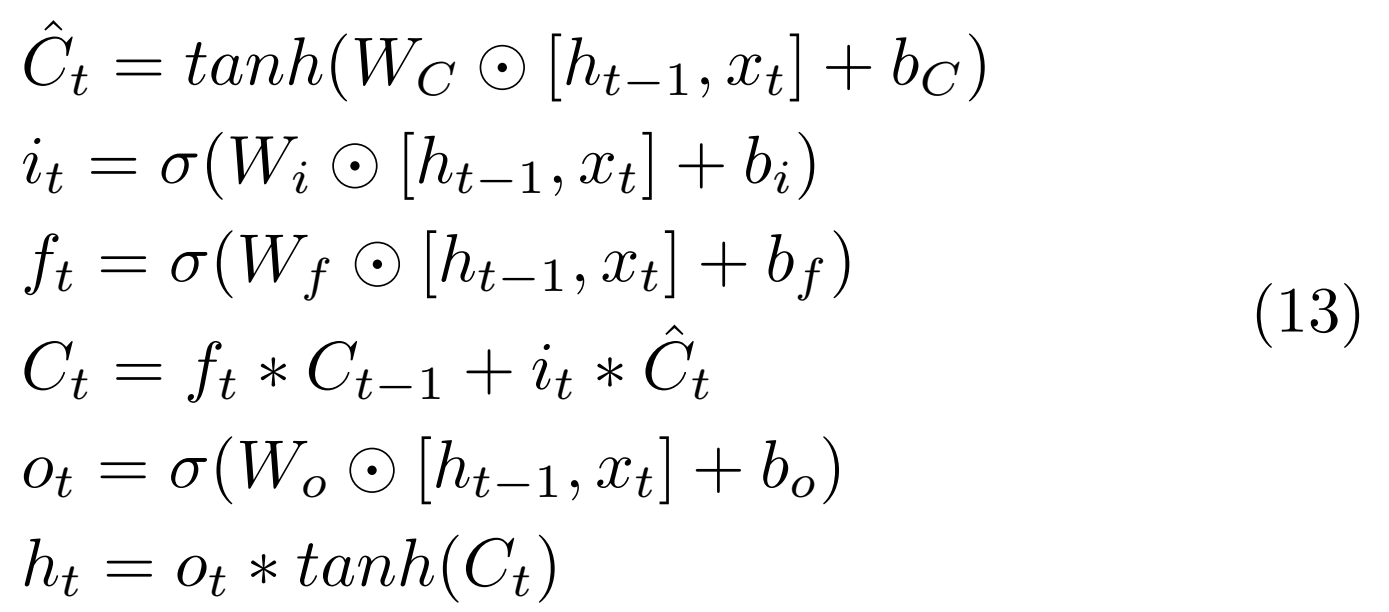## 多行公式无编号

% 多行公式无编号
\begin{align*}
\hat{C}_t &= tanh(W_C\odot[h_{t-1},x_t]+b_C) \\
i_t&=\sigma(W_i\odot[h_{t-1},x_t]+b_i) \\
f_t&=\sigma(W_f\odot[h_{t-1},x_t]+b_f) \\
C_t&=f_t * C_{t-1}+i_t * \hat{C}_t \\
o_t&=\sigma(W_o\odot[h_{t-1},x_t]+b_o) \\
h_t&=o_t * tanh(C_t)
\end{align*}

实现效果：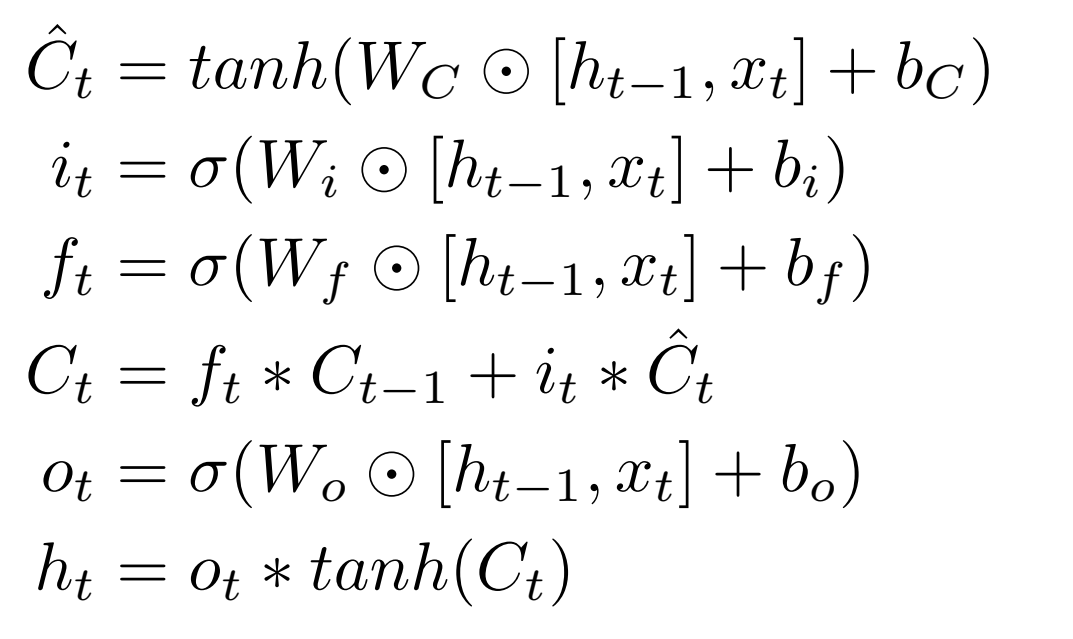## 多行公式多编号，有的行没有编号

% 多行公式多编号，有的行没有编号
\begin{align}
\hat{C}_t &= tanh(W_C\odot[h_{t-1},x_t]+b_C) \\
i_t&=\sigma(W_i\odot[h_{t-1},x_t]+b_i)  \nonumber \\
f_t&=\sigma(W_f\odot[h_{t-1},x_t]+b_f) \\
C_t&=f_t * C_{t-1}+i_t * \hat{C}_t \\
o_t&=\sigma(W_o\odot[h_{t-1},x_t]+b_o) \\
h_t&=o_t * tanh(C_t)
\end{align}

实现效果：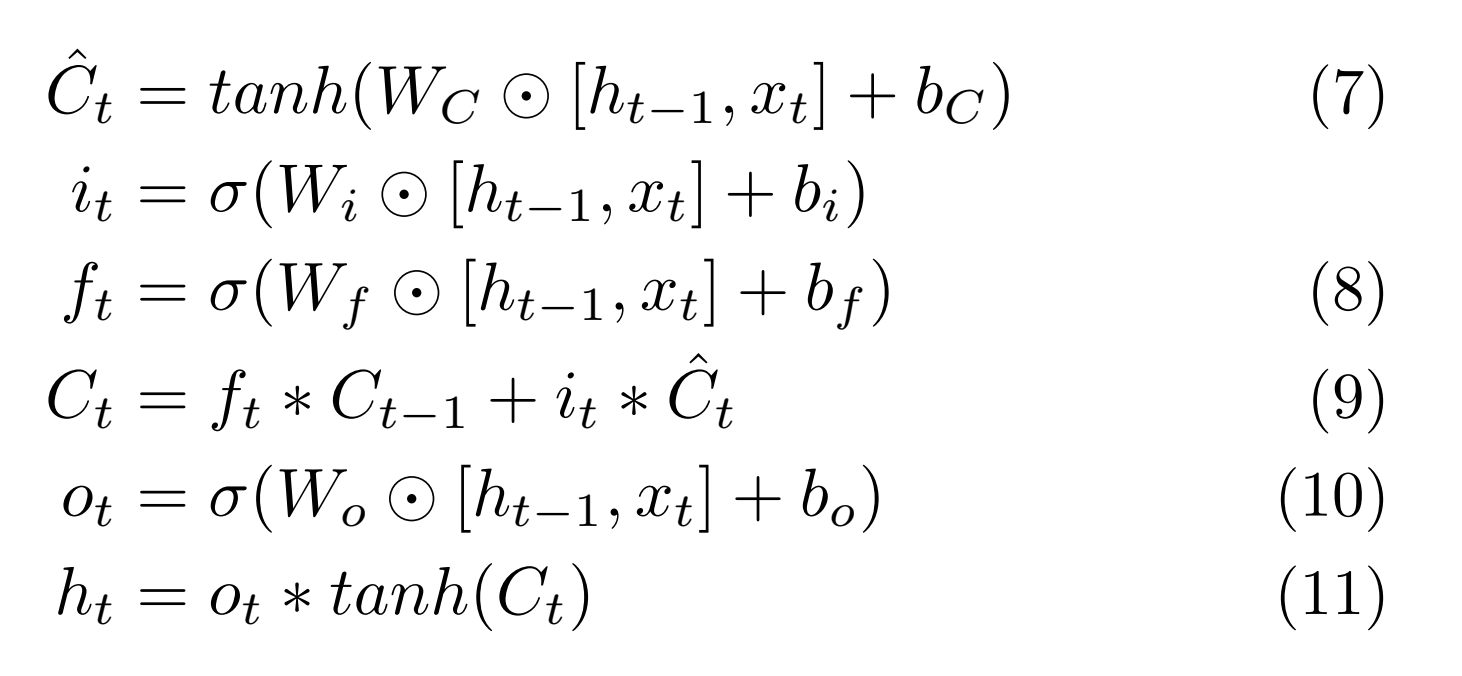## 一行公式分多行写

%split环境（用对齐）(一个公式分为多行排版) \begin{equation} \begin{split} \cos 2x &= \cos^2 x - \sin^2 x\\ &= 2\cos^2 x - 1 \end{split} \end{equation} 实现效果：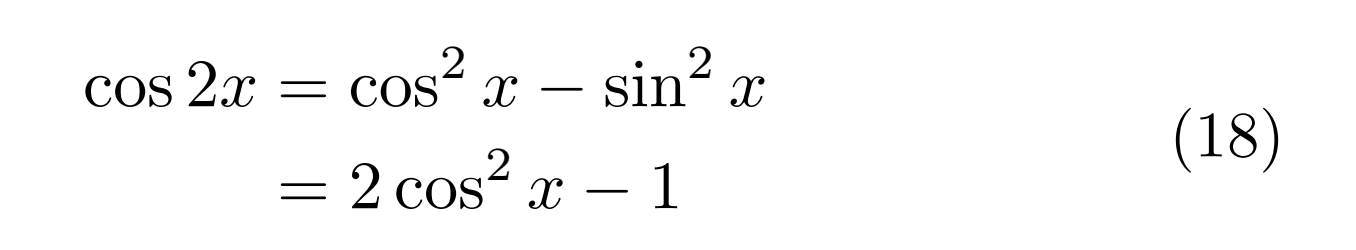## 情况（case）划分 \begin{equation} D(x) = \begin{cases} 1, & if \ x \in Q \\ 0, & if \ x \in R \end{cases} \end{equation} 实现效果：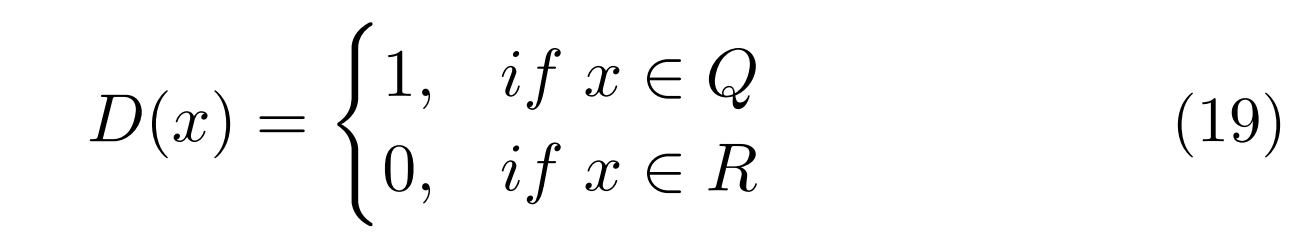## 大括号单编号 \begin{align} \left\{ %在equation环境下使用，用\left\{命令添加左大括号，用\right.以打点.结束 \begin{aligned} x&=eq1\\ y&=eq2+1 \end{aligned} \right. \end{align} 实现效果：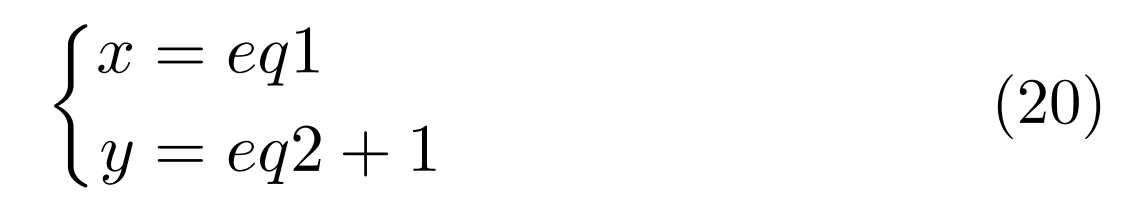## 大括号多编号 \usepackage{cases} % 大括号多编号 \begin{numcases}{} x_1&=eq1 \label{eqsystem1} \\ x_2+1&=eq2 \label{eqsystem2} \end{numcases} 实现效果：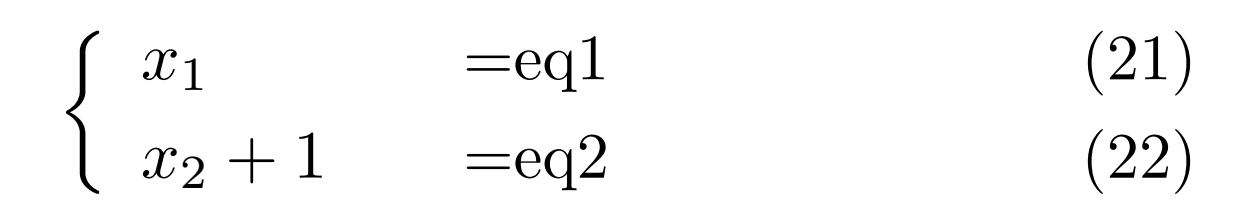更多大括号与编号的情况参考：latex多行公式加大括号、整体编号及多行编号及不同方法的区别 展开全文• 本文简单介绍一下利用WORD中公式编辑器进行公式编辑时如何编辑多行公式以及多行公式之间如何对齐的小伎俩。 目录 0. 前言 1. 公式编辑换行 2. 多行公式的对齐 # 0. 前言 本文简单介绍一下利用WORD中公式编辑器进行公式编辑时如何编辑多行公式以及多行公式之间如何对齐的小伎俩。 # 1. 公式编辑换行 首先是多行公式的编辑。 在WORD公式编辑器中，编辑完一行，如果直接按回车的话就退出公式编辑回到文本编辑状态了。如果要继续编辑多行公式的话，要按“Shift + Enter”，这样公式编辑器会切换到下一行，但是仍然在公式编辑器中【注1】。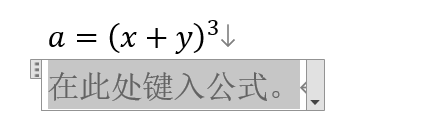如上图所示，在第一行编辑完后按“Shift+Enter”，一方面下面一行出现新的公式编辑框，另一方面，上面一行的末尾的符号是一个下箭头，而不是一个回车符。 # 2. 多行公式的对齐 其次是多行公式的对齐。 继续上面这个公式的编辑（这里只是为了示例而胡编的。。。），得到： ​​​​​​​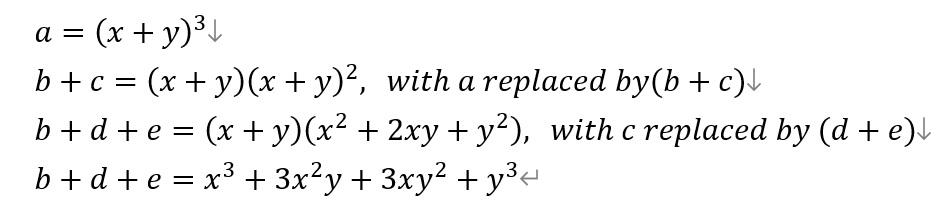显然，这个整个公式展开推导过程显得很杂乱。如果能将各行与中间的“=”为基准对齐的话就或爽心悦目得多了。 具体做法如下： （1）拖动鼠标选中整个公式 （2）点击右键会出现如下图所示的下拉菜单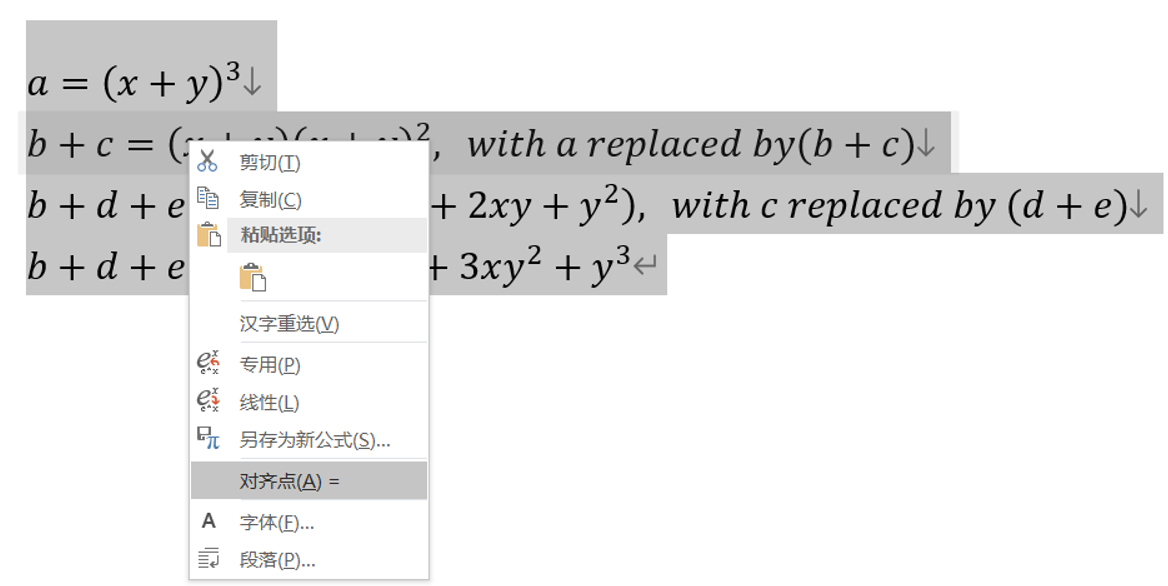（3）然后选择“对齐点(A)=” 以上操作后，得到公式如下：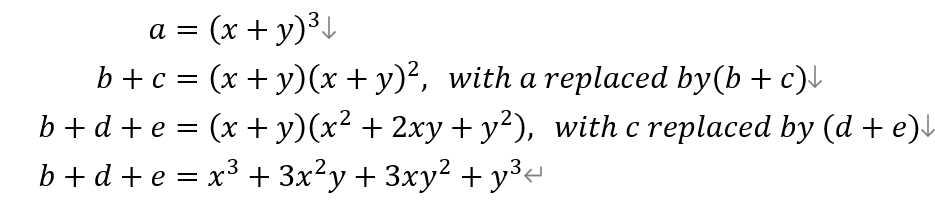展开全文经验分享 • 用 Markdown 写数学公式 ...文章介绍使用 Typora 客户端做公式笔记，主要为多行公式的 TeX\TeXTE​X 语法和公式识别两个问题。 Markdown 的用法本文不再赘述了，直接讲一下公式的问题： Remak: CSDN 使用的... # 用 Markdown 写多行公式 Markdown 是很优秀的标记语言，目前很多平台都有带 TeX \TeX 公式引擎（KaTeX, Mathjax 等）的 Markdown 编辑器。文章介绍使用 Typora 客户端做公式笔记，主要为多行公式的 TeX \TeX 语法和公式识别两个问题。 简单的 LaTeX \LaTeX 语法就不介绍了，读者如有需要可以直接拉到文章末尾看 LaTeX \LaTeX 的一份入门文档，本文直接讲一下多行公式的问题： Remak: • CSDN 使用的是 KaTeX 引擎，本文描述的 Typora 是 MathJax 引擎，渲染有所出入：1）不支持 equation 环境，2）不支持公式编号 • 源文档: Pastebin • Typora: Typora official webpage ## 行内公式和简单单行公式 行内公式： 系统状态方程\dot{x}=Ax$的解为$x=e^Ax(0)$ 渲染效果： 系统状态方程 x ˙ = A x \dot{x}=Ax 的解为 x = e A x ( 0 ) x=e^Ax(0) 简单行间公式： 系统状态方程$\dot{x}=Ax的解为 $$x=e^Ax(0)$$  渲染效果： 系统状态方程 x ˙ = A x \dot{x}=Ax 的解为 x = e A x ( 0 ) x=e^Ax(0) Remark： • 在 Typora 中，公式功能需要自行开启 • 在 Typora 中，默认是无编号，需要自行开启 ## 多行公式 ### 简单多行公式 用法：用两个$$ 嵌套，或者使用 \begin{equation}\end{equation} 示例： $$ f_1(x)\\ % 使用 \\ 换行 f_2(x)\label{Multi1} % 设置 label 是为了方便引用  \begin{equation} f_1(x)\\ f_2(x)\notag % \notag 取消编号 \end{equation} $$ 渲染效果 f 1 ( x ) f 2 ( x ) f_1(x)\\ f_2(x) f 1 ( x ) f 2 ( x ) f_1(x)\\ f_2(x) Remark: • 设置 label 可以很方便的引用公式，使用 \eqref{} 引用 • \notag 可以取消编号，相应的，\tag{} 可以设置任意的标注内容 ### array 环境 array 环境用于简单的多行公式，比普通环境多了一个对齐选项 用法：\begin{array}{l}\end{array}l 为对齐方式（left, right, center） 示例： $$ \begin{equation} \dot{\boldsymbol{x}}=f(\boldsymbol{x}) =\left\{ \begin{array}{ll} f_{1}(\boldsymbol{x}) & \boldsymbol{x} \in S_{1} \\ f_{2}(\boldsymbol{x}) & \boldsymbol{x} \in S_{2} \end{array}\right. \end{equation}  渲染效果： x ˙ = f ( x ) = { f 1 ( x ) x ∈ S 1 f 2 ( x ) x ∈ S 2 \dot{\boldsymbol{x}}=f(\boldsymbol{x}) =\left\{ \begin{array}{ll} f_{1}(\boldsymbol{x}) & \boldsymbol{x} \in S_{1} \\ f_{2}(\boldsymbol{x}) & \boldsymbol{x} \in S_{2} \end{array}\right. Remark: • \left\{\right. 用于自适应括号大小，只需要单边括号时，另一侧括号用 . 代替 • 大括号在 LaTeX \LaTeX 中用作参数括号，需要加转义字符 \ 使用 • array 环境中需要几列就设置几列的对齐方式，使用 & 分列，\\ 分行 • \boldsymbol{} 用于粗体数学符号 • array 也用于矩阵输入，但矩阵现多使用 matrix 环境 ### aligned 环境 aligned 环境用于需要设置对齐点的多行公式 用法：\begin{aligned}\end{ailgned} 示例：  \begin{equation} \begin{aligned} &\dot{\boldsymbol{x}}=A \boldsymbol{x}+B \boldsymbol{u} , \quad \boldsymbol{x}(0)=\boldsymbol{x}_{0}\\ &y=C x+D u \end{aligned} \end{equation}  渲染效果： x ˙ = A x + B u , x ( 0 ) = x 0 y = C x + D u \begin{aligned} &\dot{\boldsymbol{x}}=A \boldsymbol{x}+B \boldsymbol{u}, \quad \boldsymbol{x}(0)=\boldsymbol{x}_{0}\\ &y=C x+D u \end{aligned} Remark: • aligned 需要 amsmath 宏包支持，现在大部分的引擎都会带有该宏包 • \quad 用于生成空白间距，类似的命令有：\qquad \, \: \; （正间距）\! （负间距） • 嵌套的 equation 环境是为了生成编号 • LaTeX \LaTeX aligned 是无法单独使用的，必须嵌套在 equation 环境中，在很多其他基于 TeX \TeX 的引擎中，都默认省去了 equation 环境，任何行间公式都是当做 equaiton 环境处理，所以会出现 aligned 能够单独使用的情况，但是在需要编号的时候，aligned 是无法自动生成编号的，这时可以嵌套一个 equation 环境来生成编号 ## 简单宏定义 宏定义能够实现自定义算符、简化过于冗长的命令 自定义命令用法：\newcommand{\定义命令}[参数个数]{内容} （内容中用 #1, #2, #3 … 来设置形参） 自定义算符用法：\DeclareMathOperator{\定义命令}{算符} 示例：  \newcommand{\bx}{\boldsymbol{x}} \notag \DeclareMathOperator{\sign}{sign}  渲染效果： s i g n x , x \mathrm{sign}\,{x},\boldsymbol{x} (\sign{x},\bx) Remark: • 声明需在文章最开头设置 • 在 Typora 中，如果公式渲染异常，可以在编辑中找到 刷新公式 ## 公式识别问题 Mathpix 提供 LaTeX \LaTeX 公式识别，准确度很高，每月 50 次免费，推荐注册可以获得 50-200 次额外次数，申请 API 可以获得每月 1000 次，日常使用是足够了。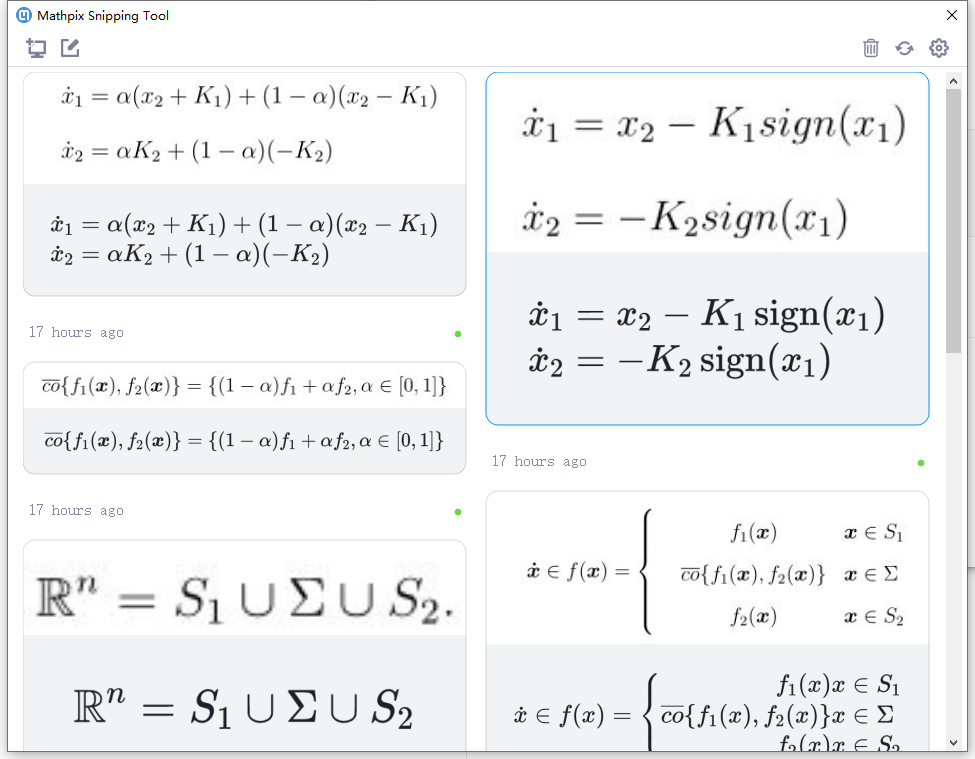## 参考文档 文章简单介绍了几个多行公式的实现，这里再贴出几个文档，以飨读者： MathJax 参考文档 LaTeX \LaTeX 参考文档 展开全文markdown latex • \begin{equation} x^n = \left\{ \begin{array}{lr} x^{n/2} \times x^{n/2} & \qquad n\%2 = 0 & \\ x \times (x^{n/2} \times x^{n/2}) & \qquad n\%2 = 0 \end{array} \right. ...python • 将几个公式罗列在一起, 是基本的产生多行公式的方法. latex为此提供了eqnarray和eqnarray环境, 用来输入按等号对其的方程组. 显示方程组的基本输入方法是使用编号的equation环境和不编号equation环境,但是里面的换行...latex • 在使用LaTeX编写论文时遇到要插入多行公式的问题 要插入的公式如下： bi1j1ai2j2={exist,i1=i2 and j1=j2;not exist,otherwise. b_{i_1j_1}a_{i_2j_2}= \begin{cases} \text{exist}, & i_1=i_...latex •经验分享 • gather虽然会居中，内部一般多行公式会分为两个编号 可以用split实现伪居中，split的本质是将&后面的公式符号进行对齐。且split环境中可以用\表示换行 会得到 这样的结果加asmath包没有用 equation不能内嵌gather ...大数据 • 公式输入：美元符号 公式 分式： \frac{分子}{分母} 开方： \sqrt[开几次方]{被开方数} 上标: X^{2} + Y^2 = Z^2 %只对^后面第一个字符处理，高次方用{}包住 下标： x_1, x_2 %使用花括号阔住复杂的下...latex • 最近在用Latex写东西，遇到了多行对齐公式的输入与编号问题，尝试了网上的许多方法，最终的解决方法记录如下： 方法一：前边\usepackage{amsmath}，利用equation，内部嵌套split，则会在公式的最后一行进行编号； ...latex • 小白之前写公式常用equation中嵌入array实现多行公式的对齐，今天找到了更简单的实现方法，分享给大家。 2.技术实现 （1）需要添加的库文件 \usepackage{amsmath} （2）实现代码 \begin{equation} \begin{...LaTex align • 基础 首先介绍内联公式和单行公式，内联公式使用单，单行公式使用双。 我定义了一个函数： y=axy =axy=...多行公式 推荐使用aligned环境，他还有个兄弟align，基本用法如下\begin{aligned} f(x) &= ax + b \latex • 一、LaTex显示（大括号连接的）多行公式/公式组合 使用cases环境实现公式的组合，&分隔公式和条件，具体LaTex代码如下：\$ D(x) = \begin{cases} \lim \limits_{x \to 0} \frac{a^x}{b+c},&x<3 \\ \pi,&...latex 经验分享
•markdown
• 1、\usepackage{amsmath} 使用amsmath包 2、equation和aligned配合使用 \begin{equation} \begin{aligned} & M_{r_{g_{s}}}(x1) \\ & N_{r_{g_{s}}} \\ \end{aligned} \end{equation} ......网络协议
• 1. 罗列多行公式 2. 拆分单个公式 3. 将公式组合成块 1. 罗列多行公式 基础语法： 1.gather 环境 ：可以在\\ 前使用\notag 命令阻止指定行不编号； \begin{gather} a + b = b + a \\ ...
• 可以使用 gather 环境实现多行公式的排版。 使用双反斜杠进行换行。 此环境不但实现了对公式的分行排版，并且对每一行公式进行了编号。 也可以使用带星号的gather环境，实现不带星号的多行公式排班，gather也可以...
• 本文的主要内容有：如何输入行内公式如何输入行间公式、多行公式常用的数学符号输入方法相信观察力敏锐的同学一定已经注意到在上一次的推送里的这段代码：\documentclass[a4paper,12pt,landscape,twocolumn]{book}\...
• Word2016创建多行公式多行公式在等号处对齐，创建自动编号和引用，多行公式居中，编号右对齐
• 源代码 \begin{equation} \begin{aligned} a+b & =1\\ c+d & =2 \end{aligned} \end{equation} 效果预览
• 1、编号在多行的中间 代码：&表示公式对齐，\\是换行 2、每一行都加编号，但是可以选择哪一行不加 代码：包\usepackage{amsmath}，\nonumber加在前面表示该行不加编号 ...
• 现在需要插入带大括号的多行公式，效果如下： LaTex编辑如下： \begin{equation} \label{eq6} [x_{i}]=\left\{ \begin{aligned} x_{ac} & , & \mu_{a}(x_{i})\geq \mu_{b}(x_{i}), \\ x_{bc} & , &...计算机视觉 深度学习 机器学习
• 但是我发现大多数人使用 Mathtype 编辑公式的效率极低，主要体现在不管啥命令都用鼠标在Mathtype 的 UI 界面中选择，这样势必会花费很多时间，因为手一会儿在键盘上，一会儿在鼠标上，很容易分心。...
• 数学方程输入对于很多理工科朋友来说是一件让人头疼的事情。不仅是数学方程本身就纷繁复杂...01LaTeX 是什么LaTeX 是一种基于 ΤΕΧ 的排版系统，具有方便而强大的数学公式排版能力。日常中大家常见的高印刷质量的...
• 第一步：创建一个三行公式 第二步：拓展为五行公式 第三步：输入五个长度不同的经典公式 第四步：用上下左右将光标控制到第一行行首，使得第一行处于全选状态 第五步：输入 &amp;amp; (按 shift + 7) 此时...
• hexo-matery多行公式（矩阵不换行）显示问题。 问题解决：不使用 mathjax，使用 Katex   这个问题是因为模板渲染引擎和 mathjax 部分字符冲突，可以选择使用Katex渲染公式。Katex是一个轻量级的快速的公式排版库。...markdown github
• 目录单独加大括号并自动加整体编号，但不能为每行编号不能加大括号的多行编号带大括号，不会自动编号也不能加编号既要大括号也要多行编号多行编号后缀 单独加大括号并自动加整体编号，但不能为每行编号 \document...latex
• LaTeX数学公式的多行公式 %导言区 \documentclass{ctexart} %ctexbook ctexrep %\usepackage{ctex} \usepackage{amsmath} \usepackage{amssymb} %正文区 \begin{document} %可以使用gather环境实现多行公式......